# Maharashtra Board Practice Set 34 Class 6 Maths Solutions Chapter 13 Profit-Loss

Balbharti Maharashtra State Board Class 6 Maths Solutions covers the Std 6 Maths Chapter 13 Profit-Loss Class 6 Practice Set 34 Answers Solutions.

## 6th Standard Maths Practice Set 34 Answers Chapter 13 Profit-Loss

Question 1.
Cost price Rs 1600, selling price Rs 2800.
Solution:
Sanju bought goods worth Rs 1600 and sold them for Rs 2800. What was his profit in percentage?
Cost price = Rs 1600, Selling price = Rs 2800
Profit = Selling price – Cost price
= 2800 – 1600
= Rs 1200
Let Sanju make profit of x%.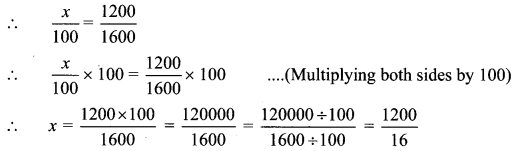∴ x = 75%
∴ Sanju made a profit of 75%.

Question 2.
Cost price Rs 2000, selling price Rs 1900.
Solution:
Rakhi bought books worth Rs 2000 and sold them for Rs 1900. What was her loss in percentage?
Cost price = Rs 2000, Selling price = Rs 1900
Loss = Cost price – Selling price .
= 2000 – 1900
= Rs 100
Let Rakhi incur a loss of x%.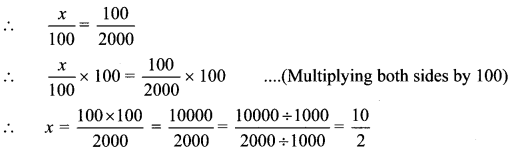∴ x = 5%
∴ Rakhi suffered a loss of 5%.

Question 3.
Cost price of 8 articles is Rs 1200 each, selling price Rs 1400 each.
Solution:
Pallavi bought 8 tables for Rs 1200 each and sold them for Rs 1400 each. What was the percentage of her profit or loss?
Cost price of 1 table = Rs 1200
∴ Cost price of 8 tables = 1200 x 8 = Rs 9600
Selling price of 1 table = Rs 1400
∴ Selling price of 8 tables = 1400 x8 = Rs 11200
Selling price is greater than the cost price.
∴ Profit = Selling price – Cost price
= 11200 – 9600
= Rs 1600
Let Pallavi make a profit of x%.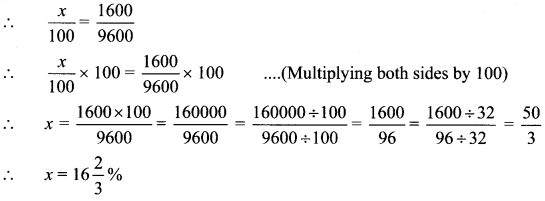∴ Pallavi made a profit of $$16\frac { 2 }{ 3 }$$ %.

Question 4.
Cost price of 50 kg grain Rs 2000, Selling price Rs 43 per kg.
Solution:
Ramesh bought 50 kg grains for Rs 2000 and sold it at the rate of Rs 43 per kg. Find the percentage of profit or loss.
Cost price of 50 kg grains = Rs 2000
Selling price of 1 kg grains = Rs 43
∴ Selling price of 50 kg grains= 43 x 50
= Rs 2150
Selling price is greater than the cost price.
∴ Profit = Selling price – Cost price
= 2150 – 2000
= Rs 150
Let Ramesh make profit of x%.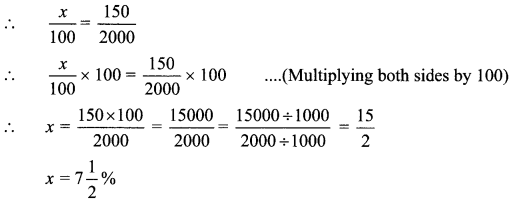∴ Ramesh made a profit of $$7\frac { 1 }{ 2 }$$ %.

Question 5.
Cost price Rs 8600, Transport charges Rs 250, Portage Rs 150, Selling price Rs 10000.
Solution:
Faruk bought a fridge for Rs 8600. He spent Rs 250 on transport and Rs 150 on portage.
If he sold the fridge for Rs 10,000, what was his percent profit or loss?
Total cost price of a fridge = Cost of fridge + Transportation cost + Portage
= 8600 + 250 + 150
= Rs 9000
∴ Selling price = Rs 10,000
Selling price is greater than the total cost price.
Profit = Selling price – Total cost price
= 10000 – 9000
= Rs 1000
Let Faruk make a profit of x% on cost price.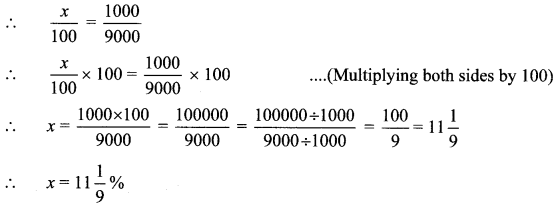∴ Faruk made a profit of $$11\frac { 1 }{ 9 }$$ %.

Question 6.
Seeds worth Rs 20500, Labour Rs 9700, Chemicals and fertilizers Rs 5600, selling price Rs 28640.
Solution:
Ramchandra bought sunflower seeds worth Rs 20500. He spent Rs 9700 on labour and Rs 5600 on chemicals and fertilizers. He sold it for Rs 28640. What is the percentage of profit or loss?
Total cost price = Cost of seeds + Labour cost + Cost of chemicals and Fertilizers
= 20500 + 9700 + 5600
= Rs 35800
Selling price = Rs 28,640
The total cost price is greater than selling price.
∴ Ramchandra suffered a loss.
Loss = Total cost price – Selling price
= 35800- 28640
= Rs 7160
Let Ramchandra incur a loss of x%.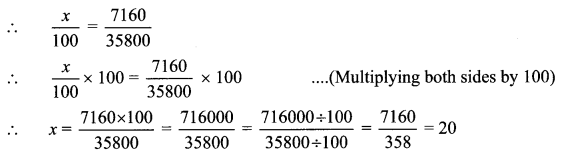∴ x = 20%
∴ Ramchandra incurred a loss of 20%.

#### Maharashtra Board Class 6 Maths Chapter 13 Profit-Loss Practice Set 34 Intext Questions and Activities

Question 1.
Maths is fun! (Textbook pg. no. 72)Arpita used 4 matchsticks to make a square. Then she took 3 more sticks and arranged them to make 2 squares. Another 3 sticks helped her to make 3 squares. How many sticks are needed to make 7 such squares in the same way? How many sticks are needed to make 50 squares?
Solution:
Matchsticks needed to make 7 squares = 4 + (6 × 3)
= 22
Matchsticks needed to make 50 squares= 4 + (49 × 3)
= 151

Question 2.
Project (Textbook pg. no. 72)
i. Relate instances of profit and loss that you have experienced. Express them as problems and solve the problems.
ii. Organize a fair. Gain the experience of selling things/trading. What was the expenditure on preparing or obtaining the good to be sold? How much were the sales worth? Write a composition about it or enact this entire transaction.
Solution:
(Students should attempt the activities on their own.)